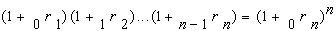﻿ 3.8 Calculating Forward Rates from Spot Ratesoffice (412) 967-9367
fax (412) 967-5958
toll-free 1 (800) 214-3480

3.8 Calculating Forward Rates from Spot Rates

The concept of a forward rate applies to any future period. Suppose you want to know what the implied forward rate (annualized) is for the period starting in Year 10 and running through to the end of Year 20. How would you calculate this?

It turns out that the general formula for computing forward rates is straightforward to derive and is constructed from the definition of a spot rate. Recall that the spot interest rate for n-periods is the geometric average of the n one period spot and forward rates:Expanding both sides by the power of n, the n-period spot rate is:and the n1 period spot rate is:The n-period forward rate is obtained by dividing the n-period spot rate raised to the power of n by the n1 period spot rate raised to the power of n-1. You can check that all terms in the product of the spot and forward rates cancel except for the period n forward rate as follows:This mathematical relationship can be generalized to provide any forward rate from the ratio of two spot rates that are raised to the power equal to their life. For example, the t period forward rate defined over Period n-t to Period t, is computed directly from the n-period spot rate and the n-t period spot rate as follows:This provides the forward rate for (n-t,n), denoted as:This result allows us to price an important financial contract that is called, not surprisingly, a foward contract.